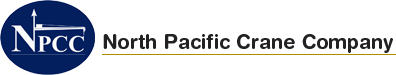### Design Optimization

### Single Telescopic Boom Analysis – for maximum tipping moment prev | next

#### SINGLE TELESCOPIC BOOM ANALYSIS – FOR MAXIMUM TIPPING MOMENT

##### 2 TON Crane
• Stress Plot :
• Max. Working Stress = 30,545 PSI
• Safety factor :
• Min. Safety Factor = 1.6
• DEFORMATION PLOT :
• Max. Deformation = 1.1 INCH
##### 5 TON Crane
• Stress Plot :
• Max. Working Stress = 16,803 PSI
• Safety factor :
• Min. Safety Factor = 1.3
• DEFORMATION PLOT :
• Max. Deformation = 0.89 IN.
##### 15 TON Crane
• Stress Plot :
• Max. Working Stress = 37,122 PSI
• Safety factor :
• Min. Safety Factor = 1.3
• DEFORMATION PLOT :
• Max. Deformation = 3.2 IN.

## MCT 15 Ton Single Tele Stress Plot

##### 20 TON Crane
• Stress Plot :
• Max. Working Stress = 24,530 PSI
• Safety factor :
• Min. Safety Factor = 2.0
• DEFORMATION PLOT :
• Max. Deformation = 1.6 INCH